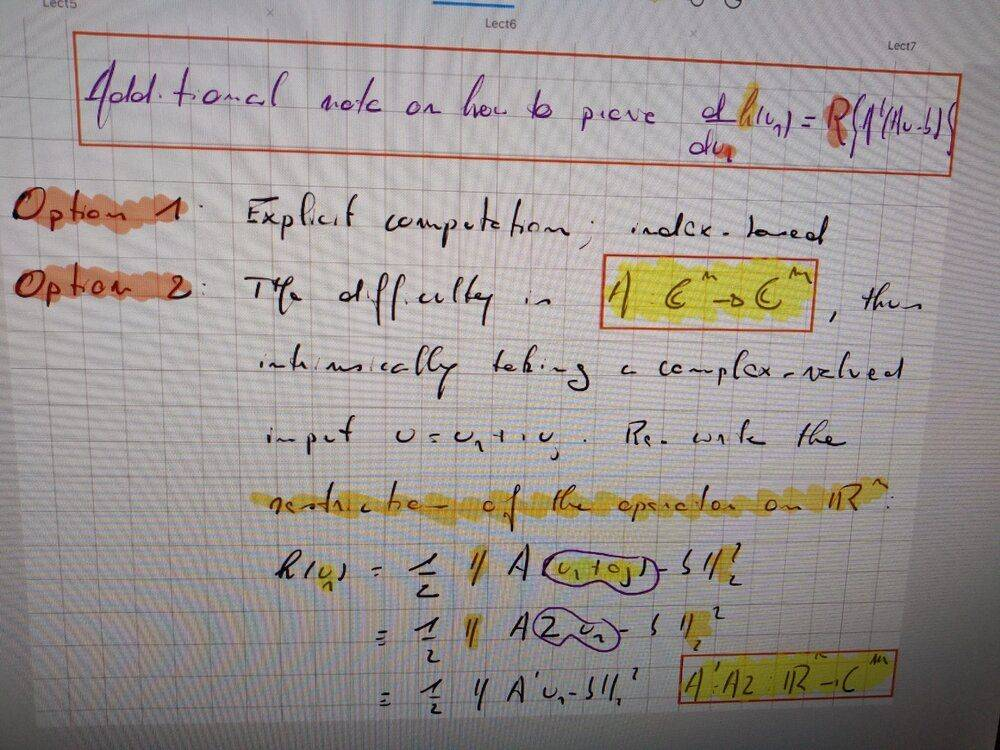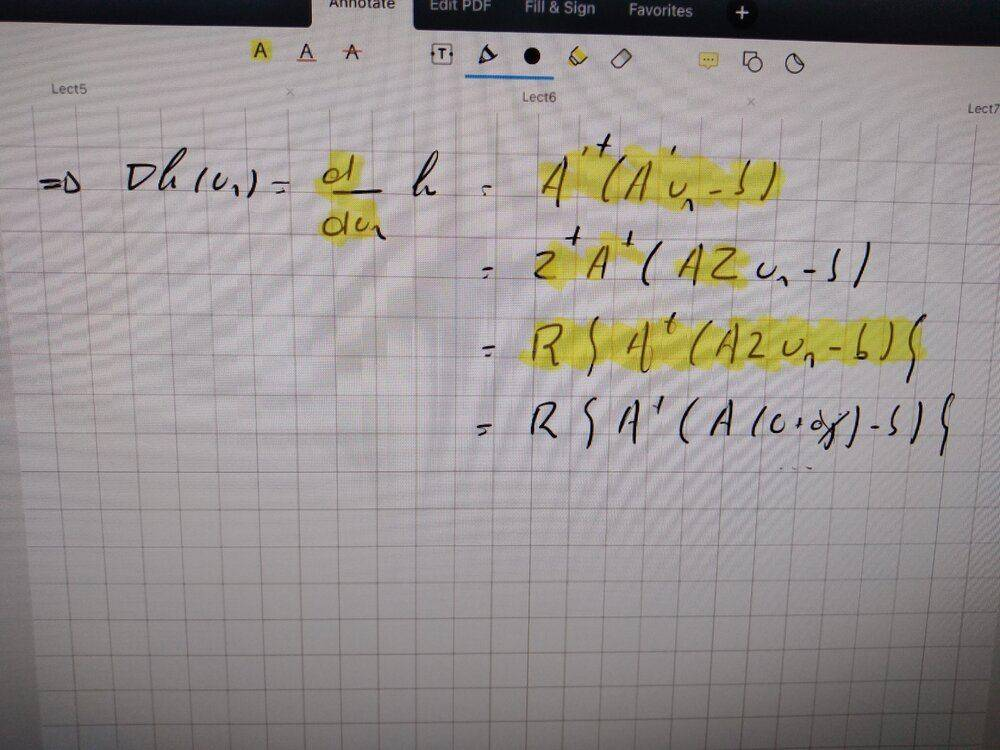# Derivative of norm of function w.r.t real-part of function

• A
• SchroedingersLion
In summary, the conversation discusses a method for showing that the partial derivative of a complex function can be rewritten as Re(A^†(Au-b)), by introducing a "zero padding" operator and interpreting Au as a function. The conversation also mentions a simpler method using matrix calculus, but there may have been errors in the calculation.

#### SchroedingersLion

TL;DR Summary
Troubles understanding an "exotic" method of taking a derivative of a norm of a complex valued function with respect to the the real part of the function.
Greetings,

suppose we have ##h(u)=\frac{1}{2} \left\|Au-b \right\|_{2}^2## with ##A## a complex matrix and ##b,u## complex vectors of suitable dimensions. Write ##u=u_1 + iu_2## with ##u_1## and ##u_2## as the real and imaginary part of ##u##, respectively.

Show that ##\frac {\partial h} {\partial u_1} = Re \left[ A^{\dagger}(Au-b) \right]##.

Now there is a straight-forward way to show this: Just rewrite ##h(u)## in terms of the matrix/vector components, take the derivative, and show that the required result is obtained. That, however, takes 2 pages of index and dagger loaded calculations.
Our lecturer presented a simpler method, but I was unable to follow his logic (there might be errors in there):He seems to interpret ##Au## as a function, and he introduces a "zero padding" operator ##\zeta## (looks like a 2), such that ##\zeta u=u_1 + i0## (?). Though I don't see why that would lead to ##A\zeta## being a function from ##\mathbb{R}^n \rightarrow \mathbb{C}^n##. Also I feel like something is wrong with the indices of ##u## here.

Note that in the case of real-valued ##A,b,u##, the derivative becomes ##A^{\dagger}(Au-b)##, which he seems to have used at the start of image 2.

Can anyone make sense of the notes?

Best,
SL

I don't understand what he is doing, but ##h(u)=\frac{1}{2} \left\|Au-b \right\|_{2}^2 = \frac 1 2 (Au-b)^T(Au-b)^*## and therefore
$$\frac {\partial h} {\partial u_1} = \frac 1 2 (\frac {\partial Au} {\partial u_1})^T(Au-b)^* + \frac 1 2 (Au-b)^T(\frac {\partial Au} {\partial u_1})^*$$
$$= \frac 1 2 \left(\frac {\partial u}{\partial u_1}\right)^T A^T (Au-b)^*+ \frac 1 2 (Au-b)^T A^* (\frac {\partial u} {\partial u_1})^*$$
Now ##\frac {\partial u} {\partial u_1}## and its complex conjugate are simply 1:
$$\frac {\partial h} {\partial u_1} =\frac 1 2 A^T (Au-b)^*+ \frac 1 2 (Au-b)^T A^*$$
$$=\frac 1 2 \left( A^\dagger (Au-b)\right)^* + \frac 1 2 (A^\dagger(Au-b))^T \stackrel{?}{=} Re(A^\dagger (Au-b))$$
Hmm... something went wrong mixing up row and column vectors. Probably from assuming ##\frac {\partial u} {\partial u_1} = 1##. But it certainly looks like this approach works.

•SchroedingersLion
mfb said:
I don't understand what he is doing, but ##h(u)=\frac{1}{2} \left\|Au-b \right\|_{2}^2 = \frac 1 2 (Au-b)^T(Au-b)^*## and therefore
$$\frac {\partial h} {\partial u_1} = \frac 1 2 (\frac {\partial Au} {\partial u_1})^T(Au-b)^* + \frac 1 2 (Au-b)^T(\frac {\partial Au} {\partial u_1})^*$$
$$= \frac 1 2 \left(\frac {\partial u}{\partial u_1}\right)^T A^T (Au-b)^*+ \frac 1 2 (Au-b)^T A^* (\frac {\partial u} {\partial u_1})^*$$
Now ##\frac {\partial u} {\partial u_1}## and its complex conjugate are simply 1:
$$\frac {\partial h} {\partial u_1} =\frac 1 2 A^T (Au-b)^*+ \frac 1 2 (Au-b)^T A^*$$
$$=\frac 1 2 \left( A^\dagger (Au-b)\right)^* + \frac 1 2 (A^\dagger(Au-b))^T \stackrel{?}{=} Re(A^\dagger (Au-b))$$
Hmm... something went wrong mixing up row and column vectors. Probably from assuming ##\frac {\partial u} {\partial u_1} = 1##. But it certainly looks like this approach works.

##h(u)=\frac{1}{2} \left\|Au-b \right\|_{2}^2 = \frac 1 2 (Au-b)^{*T}(Au-b)## ?

Doesn't matter where you put the complex conjugation if you know the product is real. ##ab^* = (a^*b)^* = a^*b##.

•hutchphd
But you end up with a little work to do at the end, yes? Your result is correct I think.

mfb said:
I don't understand what he is doing, but ##h(u)=\frac{1}{2} \left\|Au-b \right\|_{2}^2 = \frac 1 2 (Au-b)^T(Au-b)^*## and therefore
$$\frac {\partial h} {\partial u_1} = \frac 1 2 (\frac {\partial Au} {\partial u_1})^T(Au-b)^* + \frac 1 2 (Au-b)^T(\frac {\partial Au} {\partial u_1})^*$$
$$= \frac 1 2 \left(\frac {\partial u}{\partial u_1}\right)^T A^T (Au-b)^*+ \frac 1 2 (Au-b)^T A^* (\frac {\partial u} {\partial u_1})^*$$
Now ##\frac {\partial u} {\partial u_1}## and its complex conjugate are simply 1:
$$\frac {\partial h} {\partial u_1} =\frac 1 2 A^T (Au-b)^*+ \frac 1 2 (Au-b)^T A^*$$
$$=\frac 1 2 \left( A^\dagger (Au-b)\right)^* + \frac 1 2 (A^\dagger(Au-b))^T \stackrel{?}{=} Re(A^\dagger (Au-b))$$
Hmm... something went wrong mixing up row and column vectors. Probably from assuming ##\frac {\partial u} {\partial u_1} = 1##. But it certainly looks like this approach works.
Thanks mfb. I did not try to solve it using matrix calculus like this. I shall try to spot the misstep in the calculation so that it works out in the end. Originally, I started similarly, but I rewrote the scalar product ##(Au-b)^{\dagger}(Au-b) ## in terms of a sum over the components which became a very tedious calculation...

However, I would still like to understand that magic that the lecturer performed...